NEET  >  Short & Long Answer Questions: Waves - 2

# Short & Long Answer Questions: Waves - 2 - Notes | Study Physics Class 11 - NEET

 1 Crore+ students have signed up on EduRev. Have you?

Q.51 Why is the note produced by a open organ pipe sweeter than that produced by the closed organ pipe?

Answer: The note produced by open organ pipe consists of both odd and even harmonics but the note produced by closed organ pipe consists of only the odd harmonics. Due to presence of larger number of overtones or harmonics, the note produced by the open organ pipe is sweeter.
Q.52 Why are there so many holes in a flute?

Answer: The flute is basically an open organ pipe. The location of the open end can be changed by keeping the one hole open and closing the other holes. Thus the frequency of the note produced by the flute can be changed.
Q.53 Why does the pitch of a note produced by a wooden open end pipe becomes sharper when the temperature rises?

Answer: With rise in temperature, the velocity of sound increases. The fundamental frequency of an open organ pipe is given by(v1=υ/2l). Hence with an increase in the value of υ,v1 increases and the pitch of the note becomes sharper.
Q.54 When we start filling an empty bucket with water, the pitch of sound produced goes on changing. Why?

Answer: A bucket may be regarded as a pipe closed at one end. It produces a note of frequency, v= υ/4L where v is the velocity of sound in air and L is the length of air column, which is equal to depth of water level from the open end. As the bucket is filled with water, the value of L decreases. Consequently, the frequency and hence the pitch of the sound produced goes on changing.
Q.55 A vessel is placed below a water-tap. We can estimate the height of water level reached in the vessel from a distance simply by listening the sound. How?

Answer: The frequency of the note produced by an air column is inversely proportional to its length. As the level of water in the vessel rises, the length of the air column above it decreases. It produces sound of decreasing frequency i.e., the sound becomes shriller. From the extent of shrillness of sound, we can estimate the height up to which the vessel has been filled with water.
Q.56 If oil of density higher than the density of water is used in a resonance tube, how will the frequency change?

Answer: The frequency of vibration depends on the length of the air column. The liquid surface only causes the reflection of water. Hence frequency does not change if oil of density higher than that of water is used in the resonance tube.
Q.57 A vibrating string is heated to a higher temperature." What happens to the pitch of the note produced by it?

Answer: When a string is heated to a higher temperature, its length increases and its frequency of vibration decreases(v∝1/L). Hence the pitch of the note produced by it decreases.
Q.58 Why are strings of different thicknesses and materials are used in a sitar or a violin?

Answer: Fundamental frequency of a stretched string,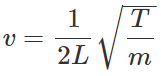When we use strings of different thicknesses and materials, they have different values of mass per unit length (m). So the strings will produce notes of different frequencies.

Q.59 A tuning fork is in resonance with a closed pipe. But the same tuning fork cannot be in resonance with an open pipe of same length. Why?

Answer: As the tuning fork of frequency v is in resonance with a closed pipe of length L, so v= υ/4L But an open pipe of length L produces a frequency of υ/2L. Hence it cannot be in resonance with the tuning fork of frequency v(=υ/4L).
Q.60 Why does the pitch of a note produced by a wooden open end organ pipe become shaper when the temperature increases?

Answer: The fundamental frequency of an open end organ pipe is given by v= υ/2L As the temperature increases, the velocity of sound (υ) increases. The frequency v increases. The pitch of the note produced becomes sharper.
Q.61 Two organ pipes of same length open at both ends produce sound of different frequencies if their radii are different. Why?

Answer: The fundamental frequency of an open organ pipe is given by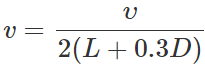Here D is the diameter of the tube and 0.3 D is the end correction. Obviously, two organ pipes of same length but of different radii will produce sounds of different frequencies.
Q.62 How does the frequency of a tunning fork change, when the temperature is increased?

Answer: As the temperature increases, the length of the prong of the tuning fork increases. This increases the wavelength of the stationary waves set up in the tuning fork. As frequency, v∝1/λ,so frequency of the tuning fork decreases.
Q.63 How does the frequency of a vibrating wire change, when the attached load is immersed in water?

Answer: When the load is immersed in water, its apparent weight decreases. So the tension in the string decreases. As v∝√T, the frequency of the vibrating wire decreases.
Q.64 What points of the stretched string between the fixed points must be plucked and touched to excite its second harmonic?

Answer: In the second harmonic, there is a node at the centre and two antinodes at 1/4 and 3/4 points. To excite second harmonic, the wire should be plucked at its one-fourth length and touched at its centre.
Q.65 What is the function of the wooden box in the sonometer? Does it increase or decrease the duration of emission?

Answer: The function of the wooden box in a sonometer is to increase the sound intensity by its forced vibrations. It decreases the duration of emission of sound energy.
Q.66 Why is a sonometer box provided with holes?

Answer: The holes in the walls of the sonometer box keep the inside air in contact with the outside air. When the sonometer wire vibrates, the vibrations are handed over from the bridges to the upper surface of the sound-board and the air inside it. Consequently, the outside air also begins to vibrate and a loud sound is heard.
Q.67 The beats are not heard, if the difference in frequencies of the two sounding notes is more than 10. Why?

Answer: If the difference in frequencies of the two waves is more than 10, we shall hear more than 10 beats per second. Due to persistence of hearing, our ear is notable to distinguish between two sounds as separate if the time interval between them is less than (1/10) th of a second. Hence beats heard will not be distinct if the number of beats produced per second is more than 10.
Q.68 Why do we not hear beats due to sound waves produced by the violins in the violin-section of an orchestra?

Answer: All the violins in the violin section of an orchestra are tuned to the same frequency. Since there is no difference in the frequencies of these violins, no beats are heard.

Q.69 As in sound, can beats be observed by two light-sources?

Answer: No, to observe beats by two light-sources the phase difference between the sources should change regularly. In light sources, however this change occurs at random, because the light-source consists of a large number of atoms and each atom emits wave independently.
Q.70 Is it necessary for beat production that the two waves must have exactly equal amplitudes?

Answer: It is not at all necessary that the amplitudes of two waves producing beats should be equal. It is only when we wish to get zero sound at minima that the two amplitudes should be equal. However, the beats become more clear as the amplitudes of two waves approach each other.
Q.71 If two sound waves of frequencies 500 Hz and 550 Hz superpose, will they produce beats? Would you hear the beats?

Answer: Yes, the sound waves will produce 50 beats every second. But due to persistence of hearing, we would not be able to hear these beats. We would hear a continuous sound of frequency 50 Hz, called beat tone.
Q.72 Can we hear beats when sounds from two different sources are heard together?

Answer: Yes. Though the two sources are not coherent, they can produce beats.
Q.73 Does Doppler's effect apply to only sound waves?

Answer: No, it applies to light waves also.
Q.74 What physical change occurs when a source of sound moves and the listener is stationary?

Answer: Wavelength of sound waves changes.
Q.75 What physical change occurs when the source of sound is stationary but the listener moves?

Q.76 Will there be Doppler effect, when the direction of motion of the source or observer is perpendicular to the direction of propagation of sound?

Answer: No, there is no Doppler effect, when the source or observer moves perpendicular to the direction of propagation of sound.
Q.77 A person riding on a merry-go-round emits a sound wave of a certain frequency. Will the person at the centre observe Doppler effect?

Answer: No, because the source moves perpendicular to the line joining the source and the observer.
Q.78 Will there be any Doppler effect, if both the sound and the listener are moving with the same velocity and in the same direction?

Answer: The apparent frequency is given by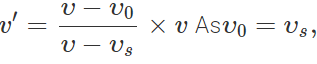therefore, v′=v i.e. there is no Doppler effect.
Q.79 What is an echo? What should be the minimum distance between the source of sound and the reflector for hearing a distinct echo?

Answer: Echo is the phenomenon of repetition of sound due to its reflection from the surface of a large obstacle. If s be the distance between the source and reflector, υ the velocity of sound and t be the total time taken by sound to reach the listener after the reflection, then 2s=υt or s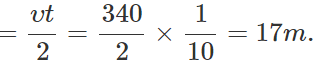Q.80 Explain why we cannot hear an echo in a small room?

Answer: For an echo of a simple sound to be heard, the minimum distance between the speaker and the walls should be 17 m. As the length of a room is generally less than 17 m, so we do not hear an echo.
Q.81 What do you mean by reverberation? What is reverberation time?

Answer: The phenomenon of persistence or prolongation of sound after the source has stopped emitting sound is called reverberation. The time for which the sound persists until it becomes inaudible is called the reverberation time.
Q.82 What is the difference between an echo and a reverberation?

Answer: An echo is produced when sound reflected from a distant obstacle comes back after an interval of 1/10 second or more. In an echo, the original and reflected sounds are heard separately. Reverberation, on the other hand, consists of successive reflections which follow each other so quickly that they cannot produce separate echoes.
Q.83 The reverberation time is larger for an empty hall than in a crowded hall. Why?

Answer: In a crowded hall, the absorption of sound waves is much more than in an empty hall because reverberation depends upon the total absorbing material in the hall.
Q.84 Thick and long curtains are preferred in a big hall. Why?

Answer: A big hall has large reverberation time due to which different syllables are not heard distinctly. By making use of thick and long curtains, which have large absorption coefficient, reverberation time can be suitably decreased.
Q.85 An organ pipe emits a fundamental note of frequency 128 Hz. On blowing into it more strongly it produces the first overtone of frequency 384 Hz. What is the type of pipe - closed or open?

Answer: The organ pipe must be a closed organ pipe, because the frequency of the first overtone is 3 times the fundamental frequency.
Q.86 What are infrasonics and ultrasonics?

Answer: Frequencies below 20 Hz are called infrasonics. Frequencies above 20,000 Hz are called ultrasonics.

Q.87 How do we identify our friend from his voice while sitting in a dark room?

Answer: On the basis of quality of sound.
Q.88 What determines the quality of sound?

Answer: Quality of sound is determined by the number of harmonic components present in the sound.
Q.89 A violin note and a sitar note may have the same frequency and yet we can distinguish between the two notes. Explain, why it is so.

Answer: This is due to the fact that overtones produced by the two sources may be different. In other words the quality of sound produced by two instruments of same fundamental frequency is different.
Q.90 What do you understand by the fidelity of an instrument?

Answer: The property of a device to reproduce the original sound in all its details is called its fidelity.
Q.91 What is the factor on which pitch of a sound depends?

Answer: The pitch of a sound depends on its frequency.
Q.92 Where will a man hear a louder sound - at the node or at the antinode in case of a stationary wave?

Answer: The sound is heard due to variation in pressure, which is given by ΔP=−Elasticity×Strain At the antinodes, amplitude is maximum but strain is minimum. At the nodes, the amplitude is minimum but strain is maximum. So variation in pressure is maximum at the nodes. Hence a loud sound is heard at node not at antinode.
Q.93 Given below are some examples of wave motion. State in each case, if the wave motion is transverse, longitudinal or a combination of both : (i) Motion of a kink in a long coil spring produced by displacing one end of the spring sideways. (ii) Waves produced in a cylinder containing a liquid by moving its piston back and forth. (iii) Waves produced by a motor boat sailing in water. (iv) Light waves travelling from the sun to the earth. (v) Ultrasonic waves in air produced by a vibrating quartz crystal.

Answer: (i) Transverse wave motion, because the vibrations of particles (kinks) of the spring are at right angles to the direction of wave propagation.

(ii) Longitudinal wave motion, because the molecules of the liquid vibrate to and fro about their mean position along the direction of propagation of the wave.

(iii) Combination of longitudinal and transverse waves, because the propeller of the molar boat cuts the water surface laterally and also pushes it in backward direction.

(iv) Transverse wave motion, because the light waves are electromagnetic waves in which electric and magnetic fields vibrate in the direction at right angle to each other and also to the direction of propagation of the wave.

(v) Ultrasonic waves produced by a quartz crystal in air are longitudinal because the molecules of air vibrate to and fro about their mean positions along the direction of propagation of wave due to vibrations of quartz crystal.
Q.94 Why is the sound produced in air not heard by a person deep inside the water?

Answer: The speed of sound in water is nearly four times the speed of sound in air. From Snell?s law of refraction,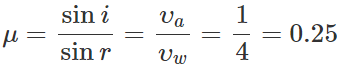For refraction, rmax=90, so (sin)max=0.25. Hence imax=14. Consequently, most of the sound produced in air and incident at ∠i>14 gets reflected back in air and very small amount is refracted into water. Hence a person deep inside water cannot hear the sound produced in air.
Q.95 In summer, the sound of a siren is heard louder in the night than in the day to a person on the earth. Why?

Answer: During the day, the temperature of the earth is maximum near the ground and it progressively decreases upwards. The velocity of sound is maximum near the ground and decreases upwards(υ∝√T). The vertical plane wave fronts produced by the siren continuously bend upwards, so the sound waves curl upwards during the day. The temperature conditions are reversed at night. The sound waves curl downwards, making the sound of siren louder on the earth.
Q.96 What are overtones and harmonics? The presence of which makes a sound musical? Or Distinguish between harmonics and overtones.

Answer: A vibrating system such as a stretched string, an air column, etc., can vibrate with a number of frequencies. The mode with lowest frequency is called fundamental mode and others are called overtones. When the frequencies of overtones are exact multiples of the fundamental frequency, they are called harmonics. The fundamental itself is called first harmonic. The presence of the harmonics makes a sound musical.
Q.97 All harmonics are overtones but all overtones are not harmonics. How?

Answer: The overtones with frequencies which are integral multiples of the fundamental are called harmonics. Hence all harmonics are overtones. But overtones which are non-integral multiples of the fundamental are not harmonics.
Q.98 The fundamental frequency of a source of sound is 200 Hz and the Source produces all the harmonics. State, with reasons, with which of the following frequencies this source will resonate: 150, 200, 300 and 600 Hz?

Answer: The source will produce harmonics of frequencies 200 Hz, 400 Hz, 600 Hz, 800 Hz, etc. Clearly, the source will resonate with frequencies of 200 Hz and 600 Hz.
Q.99 An organ pipe is in resonance with a tuning fork. What change will have to be done in the length L to maintain the resonance, if (i) the temperature increases, (ii) hydrogen is filled in place of air and (iii) pressure becomes higher?

Answer: Suppose the organ pipe has both ends open. Then its fundamental frequency of vibration will be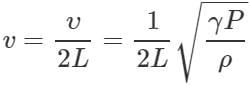(i) As the temperature increases, the velocity of sound increases, so to fix v constant, the value of L must be increased. (ii) If hydrogen is filled in the pipe in place of air, the value of density ρ decreases. To keep v constant, the value of L must be increased. (iii) The pressure has no effect on the velocity of sound. It does not affect resonance.
Q.100 A sonometer wire resonates with a tuning fork. If the length of the wire between the bridges is made twice even then it can resonate with the same tuning fork. How?

Answer: Initially, the fundamental frequency of the sonometer wire is equal to the frequency v of the tuning fork. When the length of the wire is made twice, its fundamental frequency becomes v/2. But it now produces a first overtone of frequency v, vibrating in two segments. Hence the wire can still vibrate in resonance with the tuning fork.
Q.101 Why does a tuning fork have two prongs? Would the tuning fork be of any use, if one of the prongs is cut off?

Answer: When a tuning fork is set into vibrations, its two prongs vibrate in opposite phases. However, the centre of mass of the tuning fork, which lies at the midpoint of the bend, remains at rest. Hence by holding its stem in the hand, a tuning fork can be set into vibrations and no external force is required to maintain its vibrations. If one of the prongs is cut off, the oscillations of the tuning fork will soon die out and can be maintained only with the help of an external periodic force.
Q.102 Why is a tuning fork used as a standard oscillator? On what factors does the pitch of a tuning fork depend?

Answer: When a tuning fork is struck lightly against a rubber pad, it produces only fundamental tone. If it is struck forcefully, it produces overtones which soon die out. So a tuning fork can be used as a source of standard frequency. Factors on which the pitch of a tuning fork depends:

(i) It is inversely proportional to the square of the length of its prongs. Thus v ∝ 1/l2

(ii) It is directly proportional to the thickness of the fork. v∝b

(iii) It is directly proportional to the square root of the Young's modulus of elasticity of its material. v∝√Υ

(iv) It is inversely proportional to the square root of the density of its material. v ∝ 1 /√ρ. Hence low-frequency tuning forks are long and thin while high-frequency tuning forks are short and thick.
Q.103 A sitar wire and a tabla, when sounded together, produce 5 beats per second. What can be concluded from this? If the tabla membrane is tightened, will the beat rate increase or decrease?

Answer: If v1 and v2 are the frequencies of sitar wire and the tabla membrane, then v~v2=5 If the tabla membrane is tightened i.e., tension is increased, the frequency (v2) of the sound produced by the tabla will increase. If v1>v2, the beat frequency will decrease. And if v1<v2, the beat frequency will increase.
Q.104 Doppler effect is asymmetric in sound whereas in case of light it is symmetric. Explain.

Answer: Sound waves require a material medium for their propagation. So the observed frequency of sound when the source moves towards the observer is different from the case when the observer moves towards the source with the same relative velocity. We say that the Doppler effect in sound is asymmetric. On the other hand, no material medium is required for propagation of light waves. So the apparent frequency is same whether the source moves towards the observer or the observer moves     towards the source. We say that the Doppler effect in light is symmetric.
Q.105 Distinguish between transverse and longitudinal waves.

 Transverse waves Longitudinal waves 1. The vibrations of the particles of the medium are perpendicular to the direction of  propagation of the wave. The vibrations of the particles of the medium are parallel to the direction of propagation of the wave. 2. In transverse waves, alternate crests and troughs are formed. In longitudinal waves, alternate zones of compression and rarefaction are formed. 3. These waves may be formed in solids and over liquid surfaces. These waves may be formed in solids, liquids and gases. 4. These waves do not involve changes of pressure and density of the medium. These waves involve changes of pressure and density of the medium. 5. These waves can be polarised. These waves cannot be polarised.

Q.106 What is red shift? What does it indicate?

Answer: The spectral lines received from the distant stars and galaxies are found to be shifted towards the higher wavelength side i.e., towards the red end of the visible spectrum. This shift in wavelength is called red shift. This indicates that stars and galaxies are receding away from us or the universe is expanding.
Q.107 An incident wave is represented by(x,t)=20 sin(2x−4t).Write the expression for reflected wave: (i) from a rigid boundary. (ii) from an open boundary.

Answer: (i) The wave reflected from a rigid boundary is y(x,t)=−20sin(−2x−4t)=20sin(2x+4t)

(ii) The wave reflected from an open boundary is y(x,t)=20sin(−2x−4t)=−20sin(2x+4t)
Q.108 State the principle of superposition of waves. Distinguish between conditions for the production of stationary waves and beats.

Answer: The principle of superposition states that when a number of waves travel through a medium simultaneously, the resultant displacement of any particle of the medium at any given time is equal to the algebraic sum of the displacements due to the individual waves. Mathematically, y=y1+y2+y3+.....+yn

(i) When two waves of same frequency moving with the same speed in the opposite directions in medium superpose an each other, they produce stationary waves.

(ii) When two waves of slightly different frequencies moving with the same speed in the same direction in a medium superpose on each, they produce beats.
Q.109 Differentiate between Stationary waves and Progressive waves.

 Stationary Waves Progresssive Waves (i) The disturbance remains confined to a particular region, and there is no onward motion. The disturbance travels forward, being handed over from one particle to the neighbouring particle. (ii) There is no transfer of energy in the medium. Energy is transferred in the medium along the waves. (iii) The amplitude of vibration of particles varies from zero at nodes to maximum at antinodes. The amplitude of vibration of each particle is same. (iv) The particles of the medium at nodes are permanently at rest. No particle of the medium is permanently at rest.
The document Short & Long Answer Questions: Waves - 2 - Notes | Study Physics Class 11 - NEET is a part of the NEET Course Physics Class 11.
All you need of NEET at this link: NEET

## Physics Class 11

127 videos|464 docs|210 tests
 Use Code STAYHOME200 and get INR 200 additional OFF

## Physics Class 11

127 videos|464 docs|210 tests

### How to Prepare for NEET

Read our guide to prepare for NEET which is created by Toppers & the best Teachers

Track your progress, build streaks, highlight & save important lessons and more!

,

,

,

,

,

,

,

,

,

,

,

,

,

,

,

,

,

,

,

,

,

;